# A Understanding ggplot2

Most of the recipes in this book involve the ggplot2 package, which was originally created by Hadley Wickham. It is not a part of “base” R, but it has attracted many users in the R community because of its versatility, clear and consistent interface, and beautiful output.

ggplot2 takes a different approach to graphics than other plotting packages in R. It gets its name from Leland Wilkinson’s grammar of graphics, which provides a formal, structured perspective on how to describe data graphics.

Even though this book deals largely with ggplot2, I don’t mean to say that it’s the be-all and end-all of graphics in R. For example, I sometimes find it faster and easier to inspect and explore data with R’s base graphics, especially when the data isn’t already structured properly for use with ggplot2. There are some things that ggplot2 can’t do, or can’t do as well as other plotting packages. There are other things that ggplot2 can do, but that specialized packages are better suited to handling. For most purposes, though, I believe that ggplot2 gives the best return on time invested, and it provides beautiful, publication-ready results.

Another excellent package for general-purpose plots is lattice, by Deepyan Sarkar, which is an implementation of trellis graphics. It is included as part of the base installation of R.

If you want a deeper understanding of ggplot2, read on!

## A.1 Background

In a data graphic, there is a mapping (or correspondence) from properties of the data to visual properties in the graphic. The data properties are typically numerical or categorical values, while the visual properties include the x and y positions of points, colors of lines, heights of bars, and so on. A data visualization that didn’t map the data to visual properties wouldn’t be a data visualization. On the surface, representing a number with an x coordinate may seem very different from representing a number with a color of a point, but at an abstract level, they are the same. Everyone who has made data graphics has at least an implicit understanding of this. For most of us, that’s where our understanding remains.

In the grammar of graphics, this deep similarity is not just recognized, but made central. In R’s base graphics functions, each mapping of data properties to visual properties is its own special case, and changing the mappings may require restructuring your data, issuing completely different plotting commands, or both.

To illustrate, I’ll show a graph made from the `simpledat` data set from the gcookbook package:

``````# Install gcookbook if you don't already have it installed.
# install.packages("gcookbook")

library(gcookbook)  # Load gcookbook for the simpledat data set
simpledat
#>    A1 A2 A3
#> B1 10  7 12
#> B2  9 11  6``````

The following will make a simple grouped bar plot, with the `A`s going along the x-axis and the bars grouped by the `B`s (Figure A.1):

``barplot(simpledat, beside = TRUE)``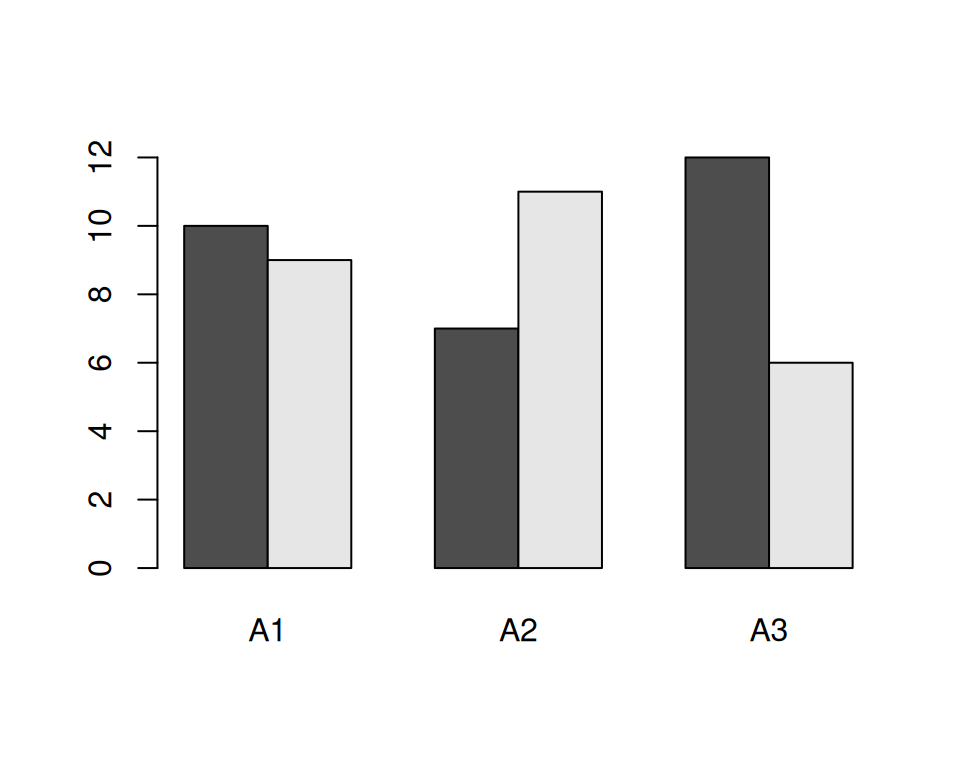Figure A.1: A bar plot made with `barplot()`

One thing we might want to do is switch things up so the Bs go along the x-axis and the As are used for grouping. To do this, we need to restructure the data by transposing the matrix:

``````t(simpledat)
#>    B1 B2
#> A1 10  9
#> A2  7 11
#> A3 12  6``````

With the restructured data, we can create the plot the same way as before (Figure A.2):

``barplot(t(simpledat), beside=TRUE)``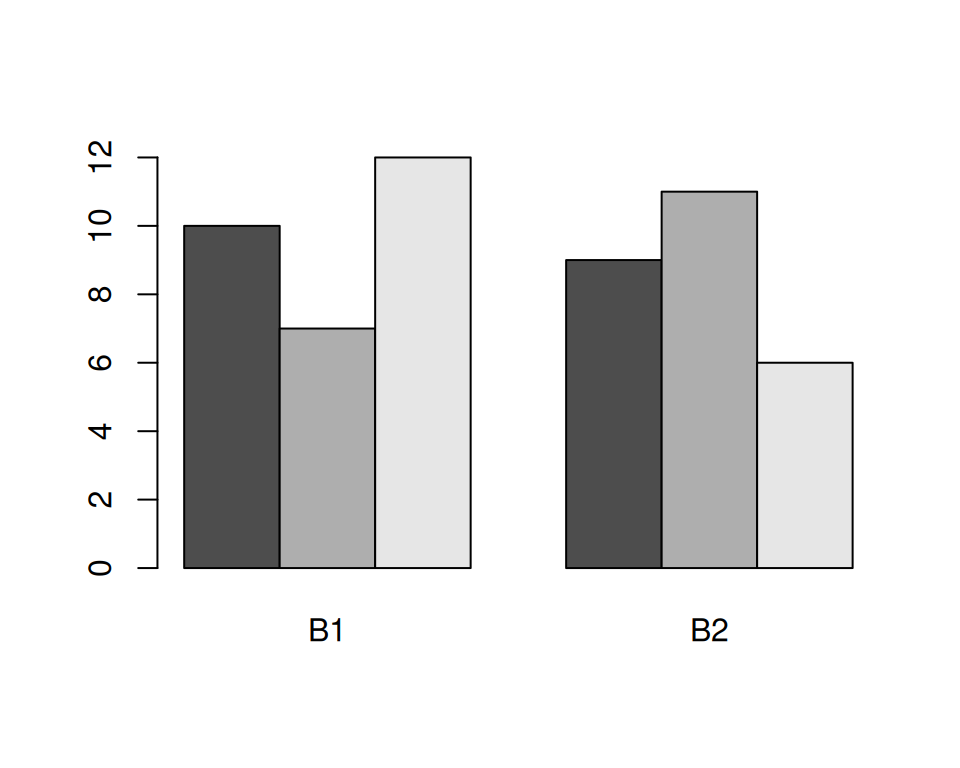Figure A.2: A bar plot with transposed data

Another thing we might want to do is to represent the data with lines instead of bars, as shown in Figure A.3. To do this with base graphics, we need to use a completely different set of commands. First we call `plot()`, which tells R to create a new plot and draw a line for one row of data. Then we tell it to draw a second row with `lines()`:

``````plot(simpledat[1,], type="l")
lines(simpledat[2,], type="l", col="blue")``````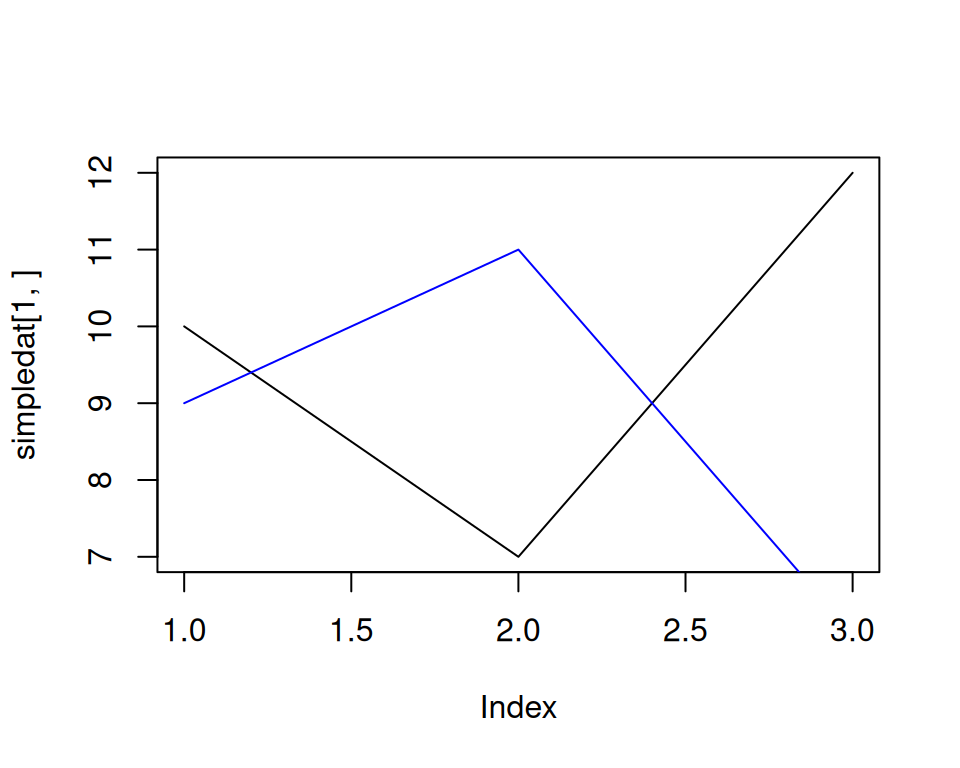Figure A.3: A line graph made with `plot()` and `lines()`

The resulting plot has a few quirks. The second (blue) line runs below the visible range, because the y range was set only for the first line, when the `plot()` function was called. Additionally, the x-axis is numbered instead of categorical.

Now let’s take a look at the corresponding code and plots with ggplot2. With ggplot2, the structure of the data is always the same: it requires a data frame in “long” format, as opposed to the “wide” format used previously. When the data is in long format, each row represents one item. Instead of having their groups determined by their positions in the matrix, the items have their groups specified in a separate column. Here is `simpledat`, converted to long format:

``````simpledat_long
#>   Aval Bval value
#> 1   A1   B1    10
#> 2   A1   B2     9
#> 3   A2   B1     7
#> 4   A2   B2    11
#> 5   A3   B1    12
#> 6   A3   B2     6``````

This represents the same information, but with a different structure. Another term for it is tidy data, where each row represents one observation. There are advantages and disadvantages to this format, but on the whole, it makes things simpler when dealing with complicated data sets. See Recipes Recipe 15.19 and Recipe 15.20 for information about converting between wide and long data formats.

To make the first grouped bar plot (Figure A.4), we first have to load the ggplot2 packag.e Then we tell it to map `Aval` to the x position, with `x = Aval`, and `Bval` to the fill color, with `fill = Bval`. This will make the `A`s run along the x-axis and the `B`s determine the grouping. We also tell it to map value to the y position, or height, of the bars, with `y = value`. Finally, we tell it to draw bars with `geom_col()` (don’t worry about the other details yet; we’ll get to those later):

``````library(ggplot2)
ggplot(simpledat_long, aes(x = Aval, y = value, fill = Bval)) +
geom_col(position = "dodge")``````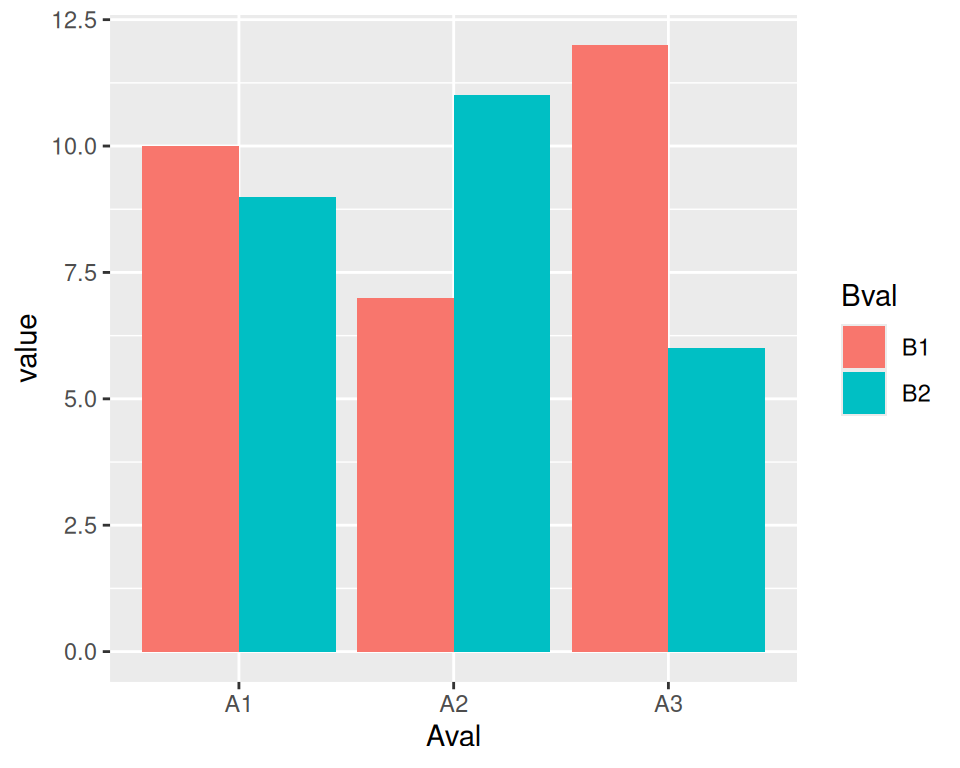Figure A.4: A bar graph made with `ggplot()` and `geom_col()`

To switch things so that the `B`s go along the x-axis and the `A`s determine the grouping (Figure A.5), we simply swap the mapping specification, with `x = Bval` and `fill = Aval`. Unlike with base graphics, we don’t have to change the data; we just change the commands for making the plot:

``````ggplot(simpledat_long, aes(x = Bval, y = value, fill = Aval)) +
geom_col(position = "dodge")``````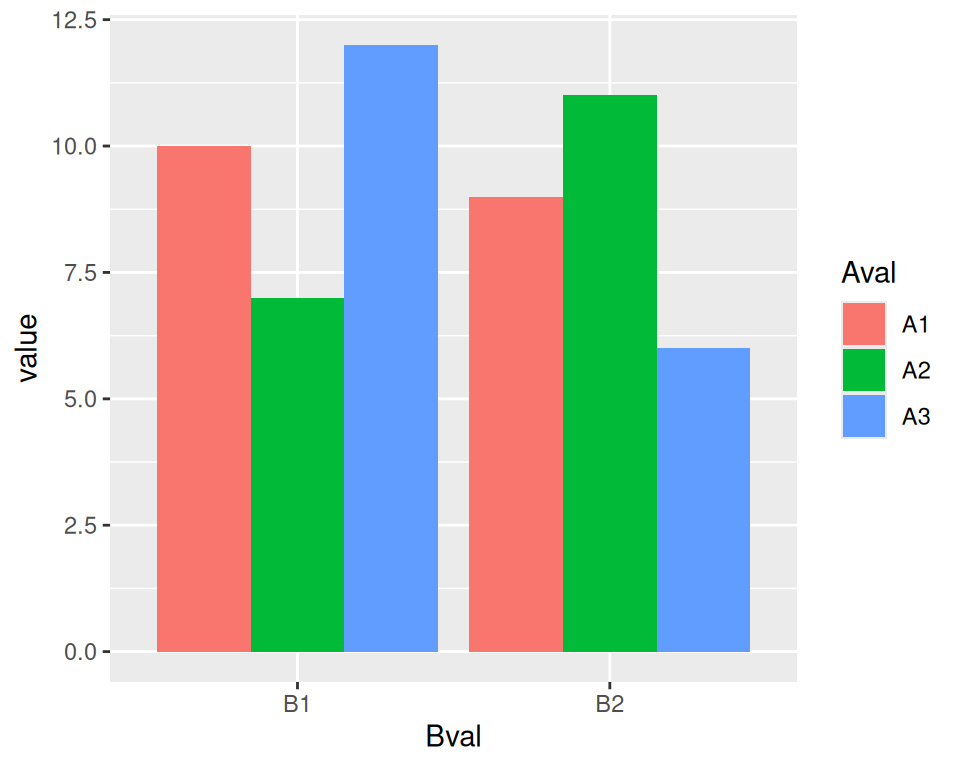Figure A.5: Bar plot of the same data, but with `x` and `fill` mappings switched

Note

You may have noticed that with ggplot2, components of the plot are combined with the `+` operator. You can gradually build up a ggplot object by adding components to it. Then, when you’re all done, you can tell it to print.

To change it to a line plot (Figure A.6), we’ll change `geom_col()` to `geom_line()`. We’ll also map `Bval` to the line color, with `colour`, instead of the fill colour (note the British spelling – the author of ggplot2 is a Kiwi). Again, don’t worry about the other details yet:

``````ggplot(simpledat_long, aes(x = Aval, y = value, colour = Bval, group = Bval)) +
geom_line()``````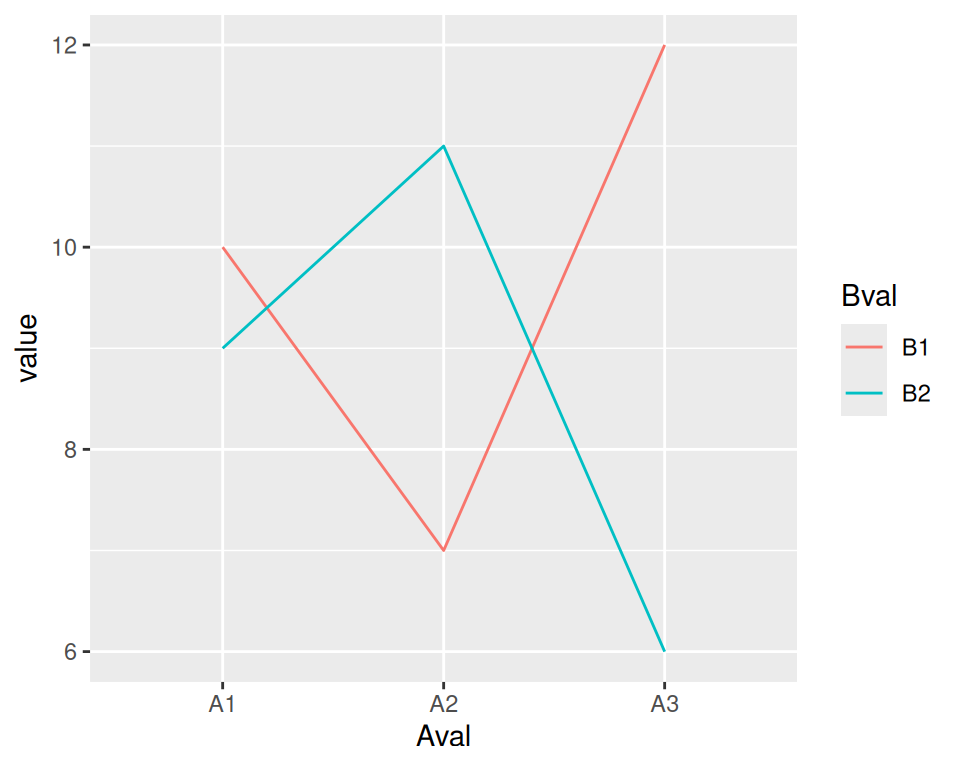Figure A.6: A line graph made with `ggplot()` and `geom_line()`

With base graphics, we had to use completely different commands to make a line plot instead of a bar plot With ggplot2, we just changed the geom from bars to lines. The resulting plot also has important differences from the base graphics version: the y range is automatically adjusted to fit all the data because all the lines are drawn together instead of one at a time, and the x-axis remains categorical instead of being converted to a numeric axis. The ggplot2 plots also have automatically-generated legends.

## A.2 Some Terminology and Theory

Before we go any further, it’ll be helpful to define some of the terminology used in ggplot2:

• The data is what we want to visualize. It consists of variables, which are stored as columns in a data frame.

• Geoms are the geometric objects that are drawn to represent the data, such as bars, lines, and points.

• Aesthetic attributes, or aesthetics, are visual properties of geoms, such as x and y position, line color, point shapes, etc.

• There are mappings from data values to aesthetics.

• Scales control the mapping from the values in the data space to values in the aesthetic space. A continuous y scale maps larger numerical values to vertically higher positions in space.

• Guides show the viewer how to map the visual properties back to the data space. The most commonly used guides are the tick marks and labels on an axis.

Here’s an example of how a typical mapping works. You have data, which is a set of numerical or categorical values. You have geoms to represent each observation. You have an aesthetic, such as y (vertical) position. And you have a scale, which defines the mapping from the data space (numeric values) to the aesthetic space (vertical position). A typical linear y-scale might map the value 0 to the baseline of the graph, 5 to the middle, and 10 to the top. A logarithmic y scale would place them differently.

These aren’t the only kinds of data and aesthetic spaces possible. In the abstract grammar of graphics, the data and aesthetics could be anything; in the ggplot2 implementation, there are some predetermined types of data and aesthetics. Commonly used data types include numeric values, categorical values, and text strings. Some commonly used aesthetics include horizontal and vertical position, color, size, and shape.

To interpret the plot, viewers refer to the guides. An example of a guide is the y-axis, including the tick marks and labels. The viewer refers to this guide to interpret what it means when a point is in the middle of the scale. A legend is another type of scale. A legend might show people what it means for a point to be a circle or a triangle, or what it means for a line to be blue or red.

Some aesthetics can only work with categorical variables, such as the shape of a point: triangles, circles, squares, etc. Some aesthetics work with categorical or continuous variables, such as x (horizontal) position. For a bar graph, the variable must be categorical-it would make no sense for there to be a continuous variable on the x-axis. For a scatter plot, the variable must be numeric. Both of these types of data (categorical and numeric) can be mapped to the aesthetic space of x position, but they require different types of scales.

Note

In ggplot2 terminology, categorical variables are called discrete, and numeric variables are called continuous. These terms may not always correspond to how they’re used elsewhere. Sometimes a variable that is continuous in the ggplot2 sense is discrete in the ordinary sense. For example, the number of visible sunspots must be an integer, so it’s numeric (continuous to ggplot2) and discrete (in ordinary language).

## A.3 Building a Simple Plot

ggplot2 has a simple requirement for data structures: they must be stored in data frames, and each type of variable that is mapped to an aesthetic must be stored in its own column. In the `simpledat` examples we looked at earlier, we first mapped one variable to the x aesthetic and another to the fill aesthetic; then we changed the mapping specification to change which variable was mapped to which aesthetic.

We’ll walk through a simple example here. First, we’ll make a data frame of some sample data:

``````dat <- data.frame(
xval = 1:4,
yval=c(3, 5, 6, 9),
group=c("A","B","A","B")
)

dat
#>   xval yval group
#> 1    1    3     A
#> 2    2    5     B
#> 3    3    6     A
#> 4    4    9     B``````

A basic `ggplot()` specification looks like this.

``ggplot(dat, aes(x = xval, y = yval))``

This creates a ggplot object using the data frame `dat`. It also specifies default aesthetic mappings within `aes()`:

• `x = xval` maps the column xval to the x position.

• `y = yval` maps the column yval to the y position.

After we’ve given ggplot the data frame and the aesthetic mappings, there’s one more critical component: we need to tell it what geometric objects to add. At this point, ggplot2 doesn’t know if we want bars, lines, points, or something else to be drawn on the graph. We’ll add `geom_point()` to draw points, resulting in a scatter plot (Figure A.7):

``````ggplot(dat, aes(x = xval, y = yval)) +
geom_point()``````

If you’re going to reuse some of these components, you canstore them in variables. We can save the ggplot object in p, and then add `geom_point()` to it. This has the same effect as the preceding code:

``````p <- ggplot(dat, aes(x = xval, y = yval))

p +
geom_point()``````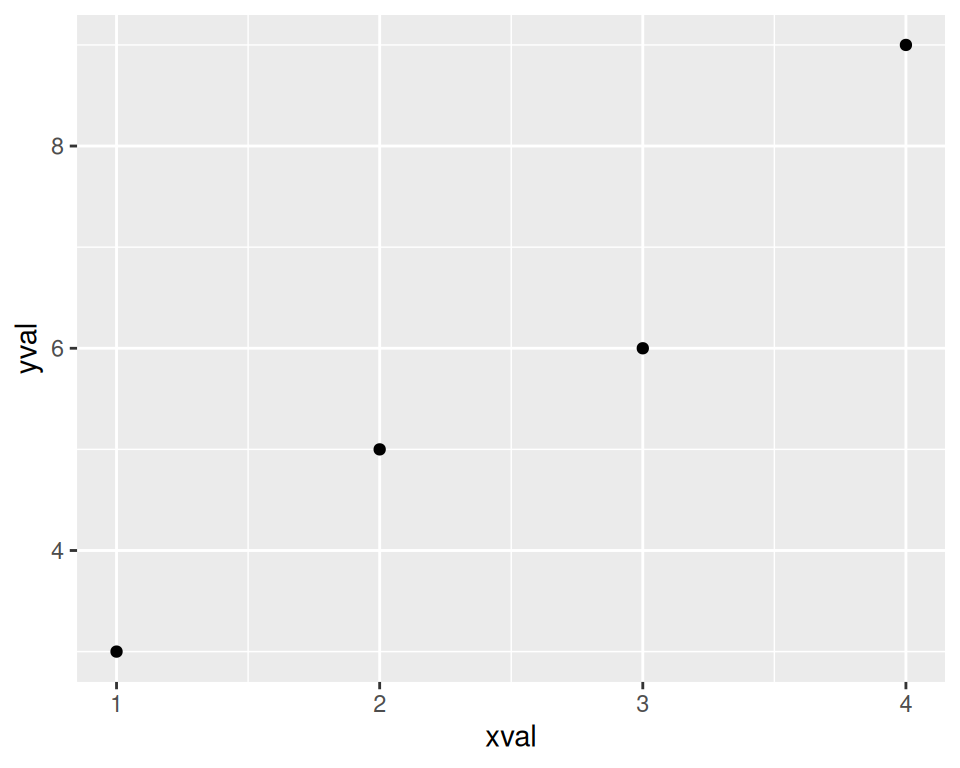Figure A.7: A basic scatter plot

We can also map the variable `group` to the color of the points, by putting `aes()` inside the call to `geom_point()`, and specifying `colour = group` (Figure A.8):

``````p +
geom_point(aes(colour = group))``````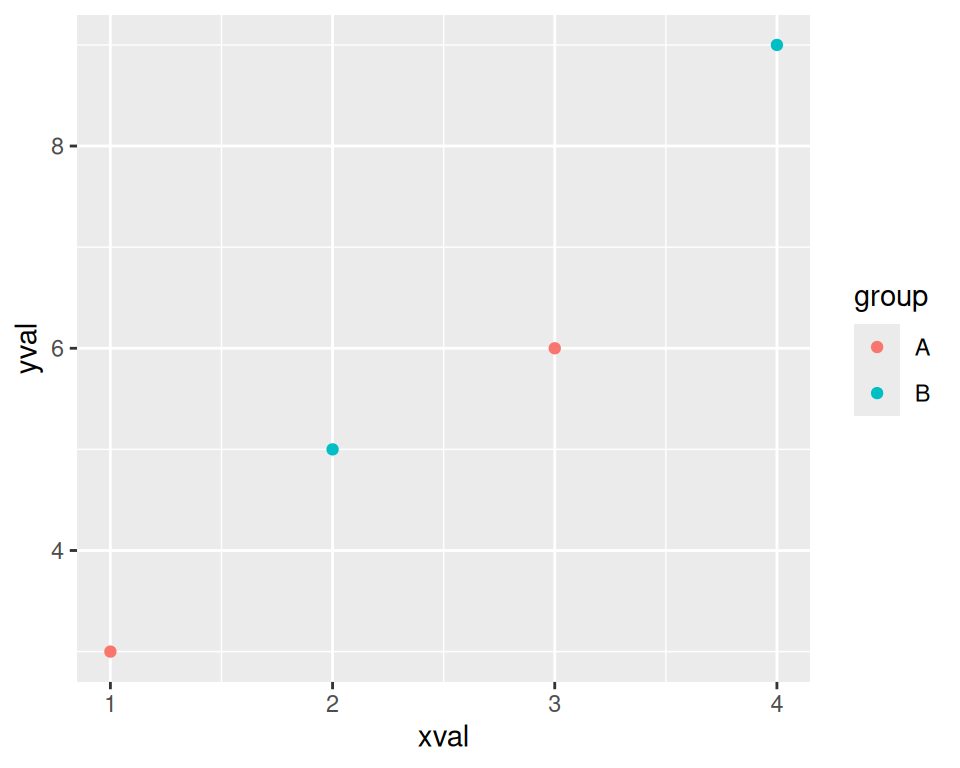Figure A.8: A scatter plot with a variable mapped to `colour`

This doesn’t alter the default aesthetic mappings that we defined previously, inside of `ggplot(...)`. What it does is add an aesthetic mapping for this particular geom, `geom_point()`. If we added other geoms, this mapping would not apply to them.

Contrast this aesthetic mapping with aesthetic setting. This time, we won’t use `aes()`; we’ll just set the value of colour directly (Figure A.9):

``````p +
geom_point(colour = "blue")``````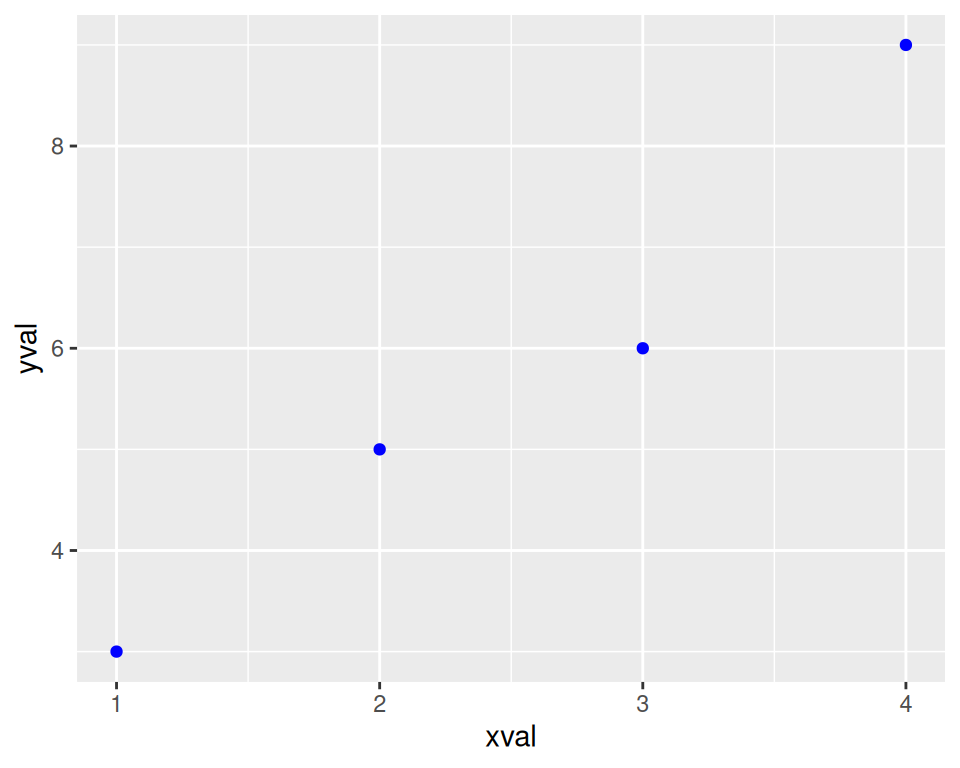Figure A.9: A scatter plot with colors set instead of mapped

We can also modify the scales; that is, the mappings from data to visual attributes. Here, we’ll change the x scale so that it has a larger range (Figure A.10):

``````p +
geom_point() +
scale_x_continuous(limits = c(0, 8))``````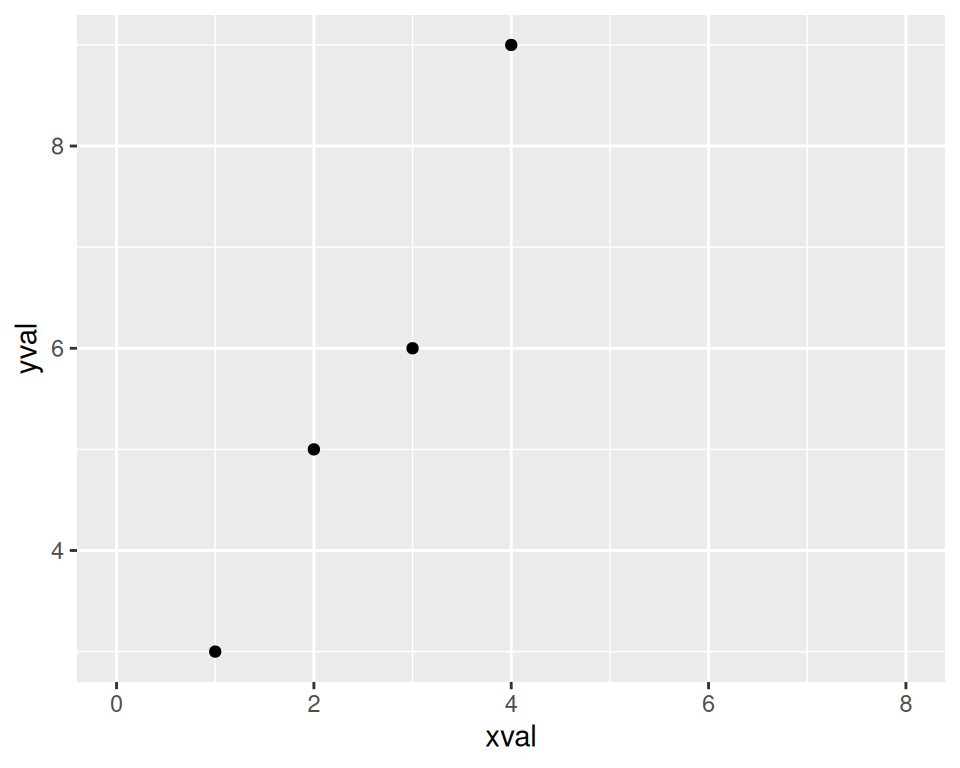Figure A.10: A scatter plot with increased x range

If we go back to the example with the `colour = group` mapping, we can also modify the color scale:

``````p +
geom_point(aes(colour = group)) +
scale_colour_manual(values = c("orange", "forestgreen"))``````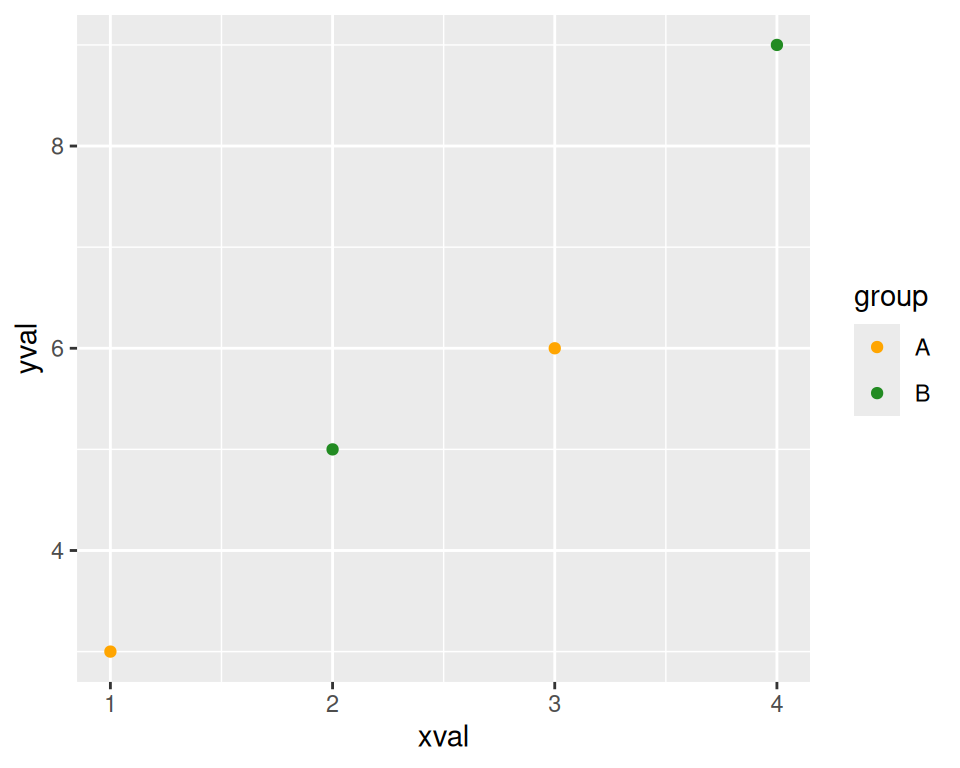Figure A.11: A scatter plot with modified colors and a different palette

Both times when we modified the scale, the guide also changed. With the x scale, the guide was the markings along the x-axis. With the color scale, the guide was the legend.

Notice that we’ve used `+` to join together the pieces. In this last example, we ended a line with `+`, then added more on the next line. If you are going to have multiple lines, you have to put the `+` at the end of each line, instead of at the beginning of the next line. Otherwise, R’s parser won’t know that there’s more stuff coming; it’ll think you’ve finished the expression and evaluate it.

## A.4 Printing

In R’s base graphics, the graphing functions tell R to draw plots to the output device (the screen or a file). ggplot2 is a little different. The commands don’t directly draw to the output device. Instead, the functions build plot objects, and the plots aren’t drawn until you use the `print()` function, as in `print(`object`)`. You might be thinking, “But wait, I haven’t told R to print anything, yet it’s made these plots!” Well, that’s not exactly true. In R, when you issue a command at the prompt, it really does two things: first it runs the command, then it calls `print()` with the result returned from that command.

The behavior at the interactive R prompt is different from when you run a script or function. In scripts, commands aren’t automatically printed. The same is true for functions, but with a slight catch: the result of the last command in a function is returned, so if you call the function from the R prompt, the result of that last command will be printed because it’s the result of the function.

Note

Some introductions to ggplot2 make use of a function called `qplot()`, which is intended as a convenient interface for making graphs. It does require a little less typing than using `ggplot()` plus a geom, but I’ve found it a bit confusing to use because it has a different way of specifying certain parameters. It’s simpler and to just use `ggplot()`.

## A.5 Stats

Sometimes your data must be transformed or summarized before it is mapped to an aesthetic. This is true, for example, with a histogram, where the samples are grouped into bins and counted. The counts for each bin are then used to specify the height of a bar. Some geoms, like `geom_histogram()`, automatically do this for you, but sometimes you’ll want to do this yourself, using various `stat_`xx functions.

## A.6 Themes

Some aspects of a plot’s appearance fall outside the scope of the grammar of graphics. These include the color of the background and grid lines in the plotting area, the fonts used in the axis labels, and the text in the plot title. These are controlled with the `theme()` function, explored in Chapter 9.

## A.7 End

Hopefully you now have an understanding of the concepts behind ggplot2. The rest of this book shows you how to use it!